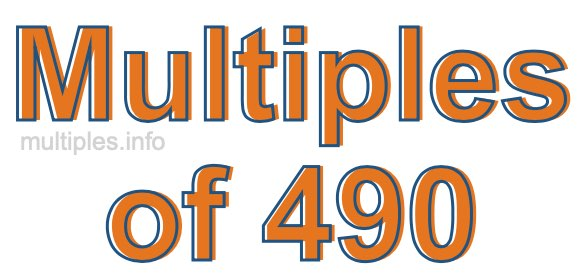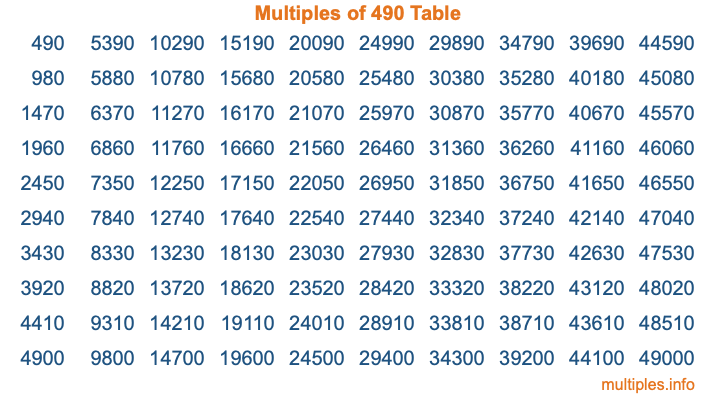Multiples of 490Welcome to the Multiples of 490 page. Here we will first teach you everything you will ever need to know about the multiples of 490, and then give you a study guide summary of everything we taught you to make sure you remember it all. Use this page to look up facts and learn information about the multiples of 490. This page will make you a multiples of four hundred ninety expert!

Definition of Multiples of 490
Multiples of 490 are all the numbers that when divided by 490 equal an integer. Each of the multiples of 490 are called a multiple. A multiple of 490 is created by multiplying 490 by an integer.

Therefore, to create a list of multiples of 490, you start with 1 multiplied by 490, then 2 multiplied by 490, then 3 multiplied by 490, and so on for as long as you want. Thus, the list of the first five multiples of 490 is 490, 980, 1470, 1960, and 2450. To see a larger list of multiples of 490, see the printable image of Multiples of 490 further down on this page. We also have a category where you can choose any nth multiple of 490.

Multiples of 490 Checker
The Multiples of 490 Checker below checks to see if any number of your choice is a multiple of 490. In other words, it checks to see if there is any number (integer) that when multiplied by 490 will equal your number. To do that, we divide your number by 490. If the the quotient is an integer, then your number is a multiple of 490.

Is  a multiple of 490?

Least Common Multiple of 490 and ...
A Least Common Multiple (LCM) is the lowest multiple that two or more numbers have in common. This is also called the smallest common multiple or lowest common multiple and is useful to know when you are adding our subtracting fractions. Enter one or more numbers below (490 is already entered) to find the LCM.

Check out our LCM Calculator if you need more details about the Least Common Multiple or if you need the LCM for different numbers for adding and subtraction fractions.

nth Multiple of 490
As we stated above, 490 is the first multiple of 490, 980 is the second multiple of 490, 1470 is the third multiple of 490, and so on. Enter a number below to find the nth multiple of 490.

th multiple of 490

Multiples of 490 vs Factors of 490
490 is a multiple of 490 and a factor of 490, but that is where the similarities end. All postive multiples of 490 are 490 or greater than 490. All positive factors of 490 are 490 or less than 490.

Below is the beginning list of multiples of 490 and the factors of 490 so you can compare:

Multiples of 490: 490, 980, 1470, 1960, 2450, etc.

Factors of 490: 1, 2, 5, 7, 10, 14, 35, 49, 70, 98, 245, 490

As you can see, the multiples of 490 are all the numbers that you can divide by 490 to get a whole number. The factors of 490, on the other hand, are all the whole numbers that you can multiply by another whole number to get 490.

It's also interesting to note that if a number (x) is a factor of 490, then 490 will also be a multiple of that number (x).

Multiples of 490 vs Divisors of 490
The divisors of 490 are all the integers that 490 can be divided by evenly. Below is a list of the divisors of 490.

Divisors of 490: 1, 2, 5, 7, 10, 14, 35, 49, 70, 98, 245, 490

The interesting thing to note here is that if you take any multiple of 490 and divide it by a divisor of 490, you will see that the quotient is an integer.

Multiples of 490 Table
Below is an image of the first 100 multiples of 490 in a table. The table is in chronological order, column by column. The first column has the first ten multiples of 490, the second column has the next ten multiples of 490, and so on.The Multiples of 490 Table is also referred to as the 490 Times Table or Times Table of 490. You are welcome to print out our table for your studies.

Negative Multiples of 490
Although not often discussed or needed in math, it is worth mentioning that you can make a list of negative multiples of 490 by multiplying 490 by -1, then by -2, then by -3, and so on, to get the following list of negative multiples of 490:

-490, -980, -1470, -1960, -2450, etc.

Multiples of 490 Summary
Below is a summary of important Multiples of 490 facts that we have discussed on this page. To retain the knowledge on this page, we recommend that you read through the summary and explain to yourself or a study partner why they hold true.

There are an infinite number of multiples of 490.

A multiple of 490 divided by 490 will equal a whole number.

490 divided by a factor of 490 equals a divisor of 490.

The nth multiple of 490 is n times 490.

The largest factor of 490 is equal to the first positive multiple of 490.

490 is a multiple of every factor of 490.

490 is a multiple of 490.

A multiple of 490 divided by a divisor of 490 equals an integer.

490 divided by a divisor of 490 equals a factor of 490.

Any integer times 490 will equal a multiple of 490.

Multiples of a Number
Here you can get the multiples of another number, all with the same attention to detail as we did for multiples of 490 on this page.

Multiples of
Multiples of 491
Did you find our page about multiples of four hundred ninety educational? Do you want more knowledge? Check out the multiples of the next number on our list!